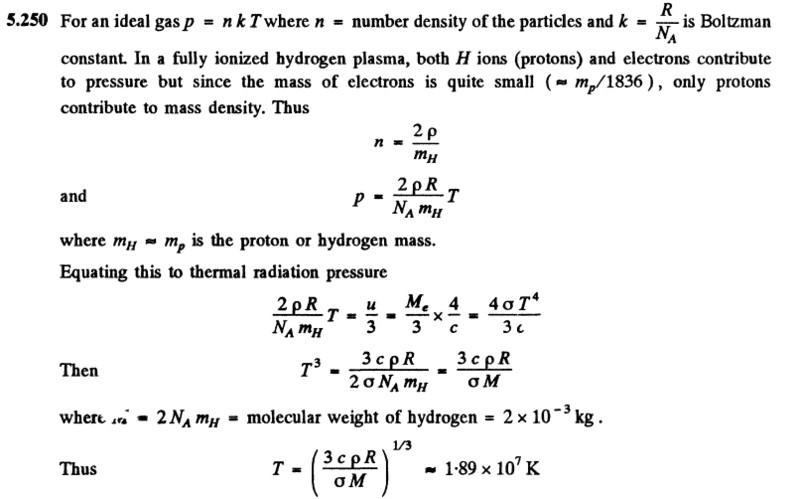# Black body radiation and gas

LCSphysicist
Homework Statement:
Find the temperature of totally ionized hydrogen plasma
of density p = 0.10 g/cm^ at which the thermal radiation pressure
is equal to the gas kinetic pressure of the particles of plasma. Take
into account that the thermal radiation pressure p = u/3, where u
is the space density of radiation energy, and at high temperatures all
substances obey the equation of state of an ideal gas.
Relevant Equations:
.The image above is the solution posted by the book. I can follow the reasoning that has been used, but i have a trouble particularly at the first equation itself.

Why should $$n = 2 \rho / m_{H}$$ instead of $$n = \rho / (2m_{H})$$, since the mass os a molecule of hydrogen is two times the hydrogen/proton mass?

If you had only protons there, the mass would be the same, and the density would be the same. From ##n=\rho / m_H## you'd get a number of protons. Now, since you know there is an extra particle for each proton that doesn't really do anything mass-wise, you get twice the number of particles that the same density/mass consideration would suggest. Unsurprisingly, ##n_{proton+electron}=2n_{proton}##.

The equation you think should be there instead suggests that you should only count half as many particles as what you get from density/mass considerations.

You could imagine some fantasy physics where each proton is accompanied by not only an electron, but also an additional imaginary particle that doesn't do anything mass-wise. The number of particles ##n## in that case should then be 3 times what you get from density/mass. Which would be ##n=3\rho / m_H##

Homework Helper
Gold Member
2022 Award
Why should $$n = 2 \rho / m_{H}$$ instead of $$n = \rho / (2m_{H})$$, since the mass os a molecule of hydrogen is two times the hydrogen/proton mass?
The number of hydrogen molecules (each of mass ##2m_H##) per unit volume is ##\frac {\rho}{2m_H}##.

One hydrogen molecule, when fully ionised, produces 4 particles (2 protons and 2 electrons).

So, after ionisation, the number of particles per unit volume is ##4 \times \frac {\rho}{2m_H} = \frac {2\rho}{m_H}##.

(Note, it’s probably better to think in terms of atoms rather than molecules for these sorts of problems.)

•LCSphysicist